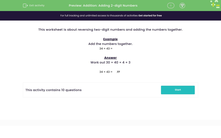### Comprehensive & curriculum aligned

In this worksheet, students add together two-digit numbers that have been reversed.Key stage:  KS 2

Curriculum topic:   Number: Addition and Subtraction

Curriculum subtopic:   Use Written Addition/Subtraction to Three Digits

Difficulty level:#### Worksheet Overview

In this activity, we will be adding together two-digit numbers.

Two-digit numbers have a tens digit and a ones digit.

To add them together, it is useful to partition each number into its tens and ones.

Let's see how this works.

34 + 43 =
 ?

To add together 34 and 43, we first partition the numbers.

34 becomes 30 and 4.

43 becomes 40 and 3

Then, we add together the tens and the ones.

30 + 40 = 70

4 + 3 = 7

Lastly, we add together the two smaller totals, to make a grand total.

70 + 7 = 77

34 + 43 =
 77

Let's try an example question together.

Example

62 + 26 =
 ?

First, we partition the numbers.

62 becomes 60 and 2.

26 becomes 20 and 6

Now, we add together the tens and ones.

60 + 20  = 80

2 + 6 = 8

Then, we add them to find the total.

80 + 8 = 88

62 + 26 =
 88

Now it's your turn to try some questions like this.

### What is EdPlace?

We're your National Curriculum aligned online education content provider helping each child succeed in English, maths and science from year 1 to GCSE. With an EdPlace account you’ll be able to track and measure progress, helping each child achieve their best. We build confidence and attainment by personalising each child’s learning at a level that suits them.

Get started